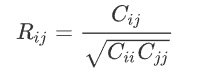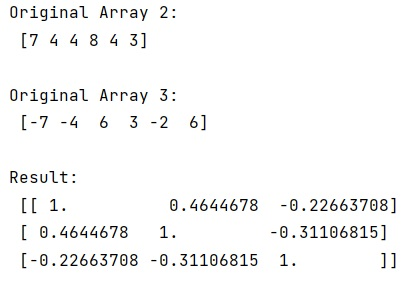# Why does corrcoef return a matrix?

Learn, why does corrcoef return a matrix in Python?
Submitted by Pranit Sharma, on January 10, 2023

NumPy is an abbreviated form of Numerical Python. It is used for different types of scientific operations in python. Numpy is a vast library in python which is used for almost every kind of scientific or mathematical operation. It is itself an array which is a collection of various methods and functions for processing the arrays.

Basically, corrcoef is used to find the correlation coefficients of two given arrays. It returns Pearson product-moment correlation coefficients.

The relationship between the correlation coefficient matrix, R, and the covariance matrix, C, isThe value of R is between -1 and 1, inclusive.

It takes an array as an argument which can be a 1-D or 2-D array containing multiple variables and observations. Each row of the array represents a variable, and each column is a single observation of all those variables.

If we pass 3 different 1-dimensional arrays in this method simultaneously, the two-data-set case becomes a special case of N-data-set class.

Let us understand with the help of an example,

## Python code to demonstrate why does corrcoef return a matrix?

```# Import numpy
import numpy as np

from numpy import *

# Creating numpy arrays
arr1 = np.array([3,6,3,6,3,2])
arr2 = np.array([7,4,4,8,4,3])
arr3 = np.array([-7,-4,6,3,-2,6])

# Display original arrays
print("Original Array 1:\n",arr1,"\n")
print("Original Array 2:\n",arr2,"\n")
print("Original Array 3:\n",arr3,"\n")

# Using corrcoef method
res = corrcoef([arr1,arr2,arr3])

# Display result
print("Result:\n",res,"\n")
```

Output: